[BACK]
 Computers, Materials & ContinuaDOI:10.32604/cmc.2022.023611Article

Optimized Energy Efficient Strategy for Data Reduction Between Edge Devices in Cloud-IoT

1Department of Computer Science and Engineering, Brainware University, Barasat, 700125, India
2Department of Computer Science and Engineering, Global Institute of Management and Technology, Krishnanagar, 741102, India
3Department of Information Technology, School of Computing Sciences, Vels Institute of Science, Technology & Advanced Studies, Chennai, 600117, India
4School of Computer Science and Engineering, SCE Taylor's University, Subang Jaya, 47500, Selangor, Malaysia
5Department of Computer Science, College of Computers and Information Technology, Taif University, Taif, 21944, Saudi Arabia
6Department of Information Technology, College of Computers and Information Technology, Taif University, Taif, 21944, Saudi Arabia
*Corresponding Author: Souvik Pal. Email: souvikpal22@gmail.com
Received: 14 September 2021; Accepted: 03 December 2021

Abstract: Numerous Internet of Things (IoT) systems produce massive volumes of information that must be handled and answered in a quite short period. The growing energy usage related to the migration of data into the cloud is one of the biggest problems. Edge computation helps users unload the workload again from cloud near the source of the information that must be handled to save time, increase security, and reduce the congestion of networks. Therefore, in this paper, Optimized Energy Efficient Strategy (OEES) has been proposed for extracting, distributing, evaluating the data on the edge devices. In the initial stage of OEES, before the transmission state, the data gathered from edge devices are supported by a fast error like reduction that is regarded as the largest energy user of an IoT system. The initial stage is followed by the reconstructing and the processing state. The processed data is transmitted to the nodes through controlled deep learning techniques. The entire stage of data collection, transmission and data reduction between edge devices uses less energy. The experimental results indicate that the volume of data transferred decreases and does not impact the professional data performance and predictive accuracy. Energy consumption of 7.38 KJ and energy conservation of 55.57 kJ was found in the proposed OEES scheme. Predictive accuracy is 97.5 percent, data performance rate was 97.65 percent, and execution time is 14.49 ms.

Keywords: Energy; efficient; internet of things; transmission; performance; cloud computing; edge devices

1  Introduction

Energy efficient transmission of cloud data is a trending research area since it has wide range of applications . The data generated using IoT devices is huge since there are wide range of IoT devices being used in the recent years. With the development of internet technology and modernization, wide ranges of devices are being used . To avoid problems faced by smart energy techniques, edge computing is employed. Edge computing helps in reducing the amount of energy involved in cloud computing like encryption, transmission, storage etc. The edge computing techniques enables the reduction in the amount of data transferred . The storage and processing of data in the cloud is done based on a distributed cloud computing infrastructure. This infrastructure is built using fog and edge computing . These applications enable the deployment of network-accessible devices . Optimization of latency is another important objective of cloud computing. Since cloud computing makes use of IoT devices that operate in real-time, these data must be processed with minimal latency. Hence, devising algorithms with minimal latency is a crucial task . The production of smart products has increased at a rapid rate in the market. Big data analytics involve the processing of data generated using smart product . Using the data processing, various actions are being automated. These automation techniques help in the reduction in human intervention . The convergence of cloud computing is another important aspect involved in the pervasive service. Connection of heterogeneous devices is possible only if the devices are compatible with each other. The establishment of compatibility is done using effective communication technologies . Indoor smart appliances are popularly being used in smart cities. To increase the reliability and efficiency of these devices, energy efficient algorithms are necessary. These smart devices are integrated based on seamless integration techniques. The wearable devices are used commonly by the elderly people . It found that even as demand for cloud computing has raised, efficiency improvements have kept energy usage almost flat across the globe's data centers.

Furthermore, studies have repeatedly shown that hyper-scale (i.e., very large) data centers use far less energy than local servers. These devices are used for monitoring the motion of the elderly . The data generated by these devices are processed using effective energy efficient algorithms, so that useful information like fall detection and abnormal action detection can be done in the case of emergencies . It is estimated that by the end of the year 2025, more than 60 billion devices will be connected using internet around the world. Thus, the big data is estimated to get bigger and bulkier. Thus, energy efficiency is the main need of the hour . To adapt to these changes mobile edge computing techniques are getting popular . Encryption is another important task in cloud computing as the cloud data is easily accessible to the public. These algorithms must be energy efficiency and at the same time more secure. Since decryption is also done at the receiver side, the key transmission must be done in a secure manner . Data processing in edge devices is done often to support the large number of IoT devices employed in smart buildings, cities and transportation. Gateways, cameras, and other IoT end devices benefit from Cloud IoT Edge's powerful data processing and machine learning capabilities, making these applications smarter, safer, and more dependable. These devices generate data at real-time. The main issue faced here is the transfer of huge amount of data. To avoid this issue, in this research, we propose a new technique for reduction of cloud data using data compression techniques. Section 2 represents the related works and Section 3 gives the paper contributions. The proposed work is discussed in Section 4 and the results are evaluated in the Section 5. The conclusion along with the future scope is given in the Section 6. Finally, the references are provided.

2  Related Works

This section discussed on related works on different strategies on energy efficiency in Cloud-IoT environment. Khan et al.  proposed a scheme for energy efficiency computation in edge computing systems. Deep reinforcement learning was employed for the energy conservation. The main advantage was the long-term power conservation due to the usage of deep reinforcement learning. However, this scheme did not provide higher performance gain. Wang et al.  did a survey on the relationship between deep learning schemes and edge computing. The applications of deep learning were discussed along with the practical implementation methodologies. Further, the fusion of edge intelligence was also presented. The usage of artificial intelligence was discussed in terms of cloud computing.

Jiang et al.  presented a scheme for edge computing based on energy aware schemes. The techniques employed for offload computation of cloud data sources were discussed along with the interactions between the edge servers. However, the methods to increase the security and encryption methodologies were not presented. Mekala et al.  presented a survey on the types of energy efficient sensors. The approaches used for green computing was presented along with the implementation techniques for the IoT devices. The difficulties involved in sensor selection and the impact of communication was analyzed. The impact rate of resource balancing was not provided in terms of mathematical modeling.

Shi et al.  designed a system for analyzing the energy-based artificial intelligence system involved in edge computing. The energy conservation was proposed to avoid the traffic congestion and energy requirements. Further, the privacy concerns involved in edge computing was discussed along with the future research directions. Kavitha et al.  presented a scheme for data management based on concurrent data. This scheme was designed for the mobile environment. The edge computing scheme was implemented in mobile platform to support delay aware systems. However, this system did not provide an optimized solution for data preservation.

Zahmatkesh et al.  presented the technologies involved in fog computing environment. An overview was provided on various technologies like machine learning, artificial intelligence and unmanned aerial vehicles employed in IoT based fog computing. However, the algorithms used for creation of energy efficient environment was not presented. Rimminen et al.  presented a virtualization model for the collaboration of edge computing. A system was designed that represented virtual nodes that were deployed over the physical devices. These devices formed the three-tier architectural framework. This paper failed to provide the methodologies for cost effective implementation.

Mohiuddin et al.  presented the security challenges faced by the cloud computing applications. The strategies used to overcome these challenges were also discussed in terms of cloud storage providers. The types of encryption algorithms employed for the security enhancement of cloud data was discussed along with their drawbacks. Authors in  proposed a scheme for the attainment of security in the low power IoT devices. The main focus was to ensure the transport layer security. This paper employed ultra-low power sensor nodes for the edge computing to increase the overall battery lifetime.

Jeyalaksshmi et al.  have discussed on mapping and allotment of VMs in cloud environment, and they have also presented reliability profile algorithm in VMs. Pal et al. [29,30] have presented resource migration algorithms in live migration concept and they have also shown the techniques to minimize execution and waiting time in cloud.

The above literature survey clearly shows that the main challenge of edge computing is energy conservation. Conservation of energy helps to increase the overall lifetime of the battery and performance of the sensor nodes. To overcome this challenge, this research provides a scheme for extracting, distributing, evaluating the data on the edge devices based on energy efficient techniques.

3  Contribution of the Paper

The contributions of this paper are as follows:

•   A novel technique called Optimized Energy Efficient Strategy (OEES) is proposed for the energy efficient data reduction between edge nodes in cloud network.

•   A new technique for data reduction between edge nodes is presented.

•   The processing of cloud IoT data is discussed using mathematical models.

The performance of the proposed scheme is analyzed using metrics like energy consumption, energy conserved, predictive accuracy, data performance rate, execution delay, cloud accuracy, edge efficiency, compression ratio and reconstruction accuracy.

4  Proposed Methodology

4.1 Proposed Optimized Energy Efficient Strategy (OEES) Model

The proposed OESS model comprises of three main layers. The lower layer is the IoT device group, the second layer is the energy conservation group, and the third layer is the cloud data center.

Fig. 1 shows the proposed OESS model. The lower layer comprises of various IoT devices that include smart home, smart factory, smart vehicles, smart transportation, smart health care centres etc. The data obtained from these devices is huge. To reduce the amount of energy required for the energy transfer, this data is reduced using the proposed OESS model. The data reduction is done in the second layer. In this layer, the data of the sensor device is processed by the edge nodes. Further, the intermediate edge nodes are also responsible for the energy efficient data reduction. The reduced data is then transferred to the cloud data centre which the top layer.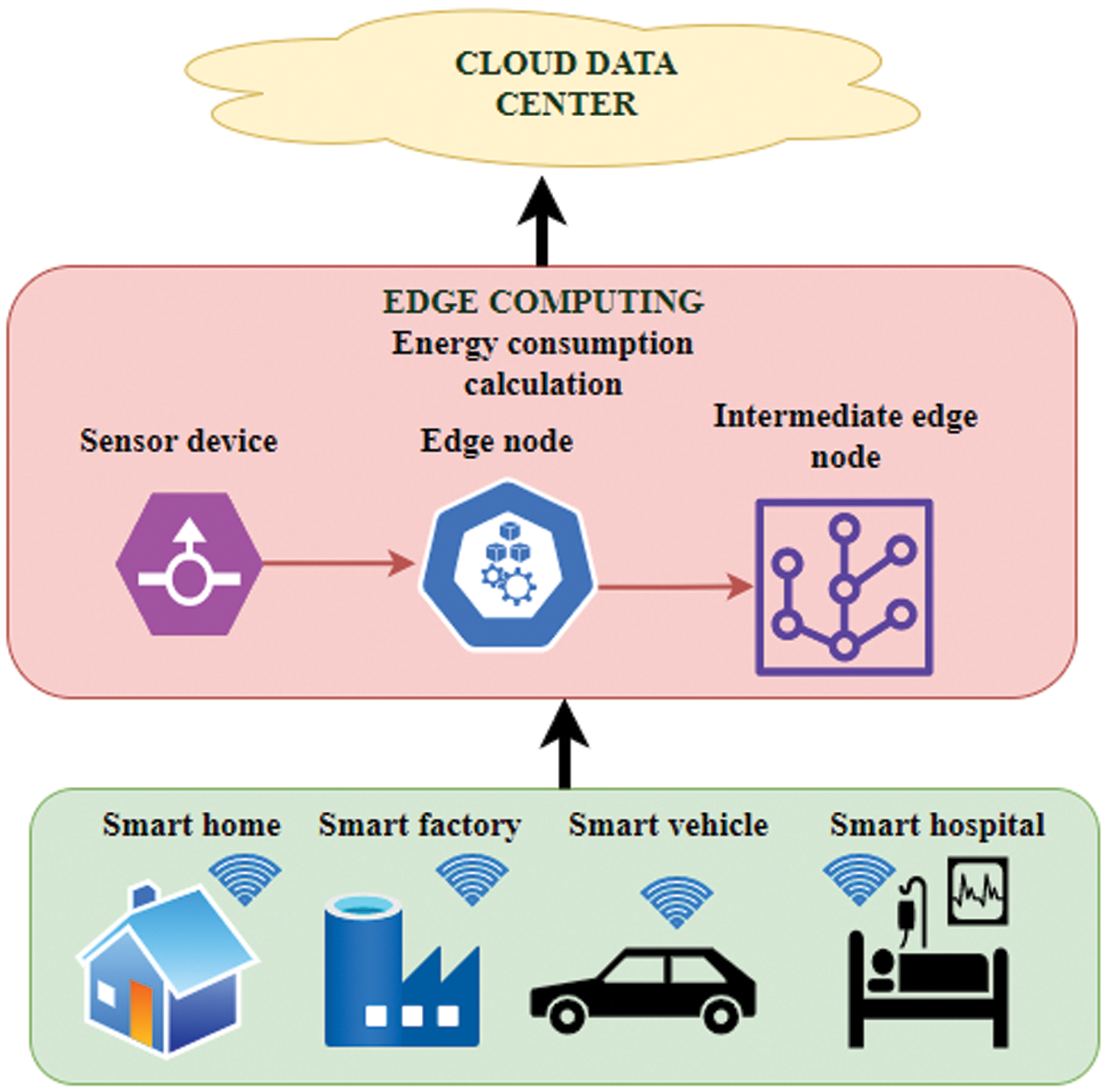Figure 1: Optimized energy efficient strategy (OEES) model

4.2 Data Reduction Between Edge Nodes

The reduction of data between the edge nodes takes place using 4 main steps. These include data reduction, data reconstruction, data processing and deep learning.

Fig. 2 shows the block diagram of data reduction between edge nodes. The data reduction is done based on compressive sensing techniques. The compression is done by the multiplication with a measurement matrix. This matrix helps in the data compression with minimal reduction in data content. The compressed data is then reconstruction using inverse measurement matrix. This matrix is constructed based on the matrix used for data compression. Finally, the compressed data is processed to make it suitable for transmission. The processed data is then transmitted to the nodes through controlled deep learning techniques.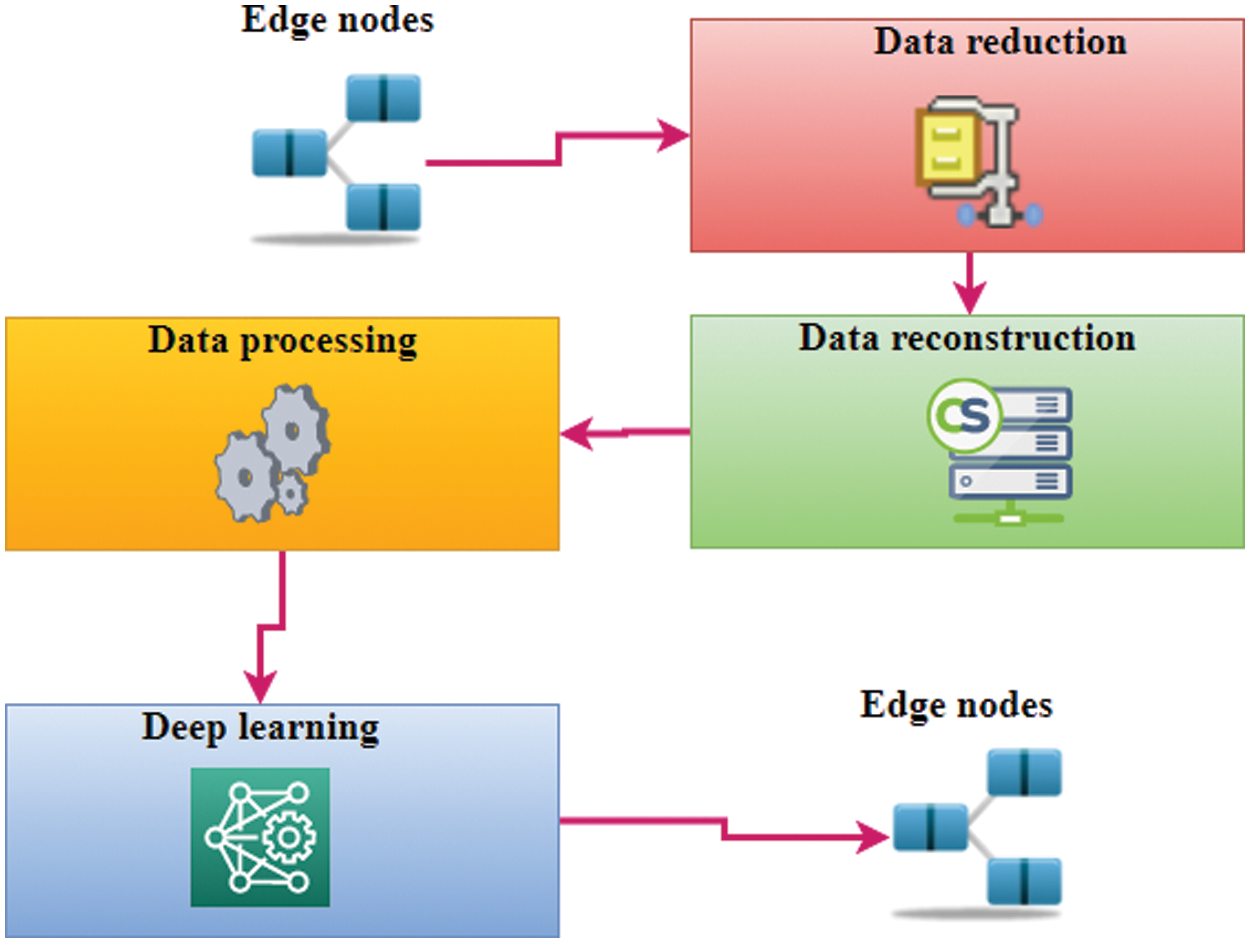Figure 2: Data reduction between edge nodes

4.3 Optimized Energy Efficient Strategy (OEES) for Data Reduction

The proposed optimized energy efficient strategy comprises of steps like data acquisition, data display, data storage, data encryption, data analysis and data compression.

Fig. 3 shows the proposed OESS model used for data reduction. In this model, the first step is the acquisition of data from the cloud-based devices. This data is initially displayed using the display modules. The cloud data is then stored for temporary usage using data storage devices. Before transmission of this data through the cloud, this data is encrypted using encryption algorithms. The encrypted data is then analyzed and compressed using the proposed OESS algorithm.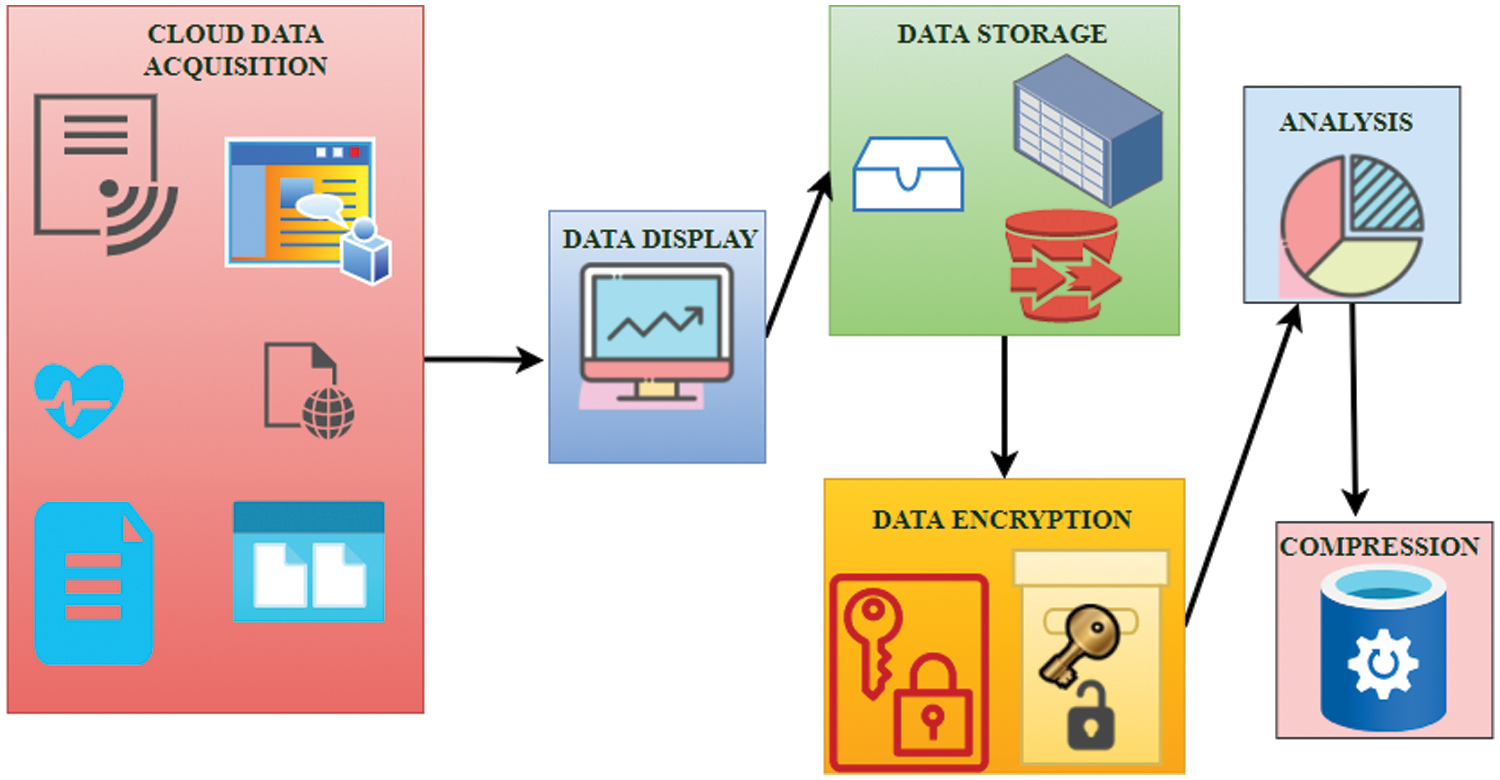Figure 3: OEES model for data reduction

This algorithm makes use of input data like task Ta={tij};i1U,j1V, energy En={eij};i1U,j1V, execution time Et={xij};i1U,j1V, virtual machine Vm={vij};i1U,j1V, time delay Td={dij};i1U,j1V, node location Nl={nij};i1U,j1V, compression rate Cr={cij};i1U,j1V and reconstruction rate Rr={rij};i1U,j1V.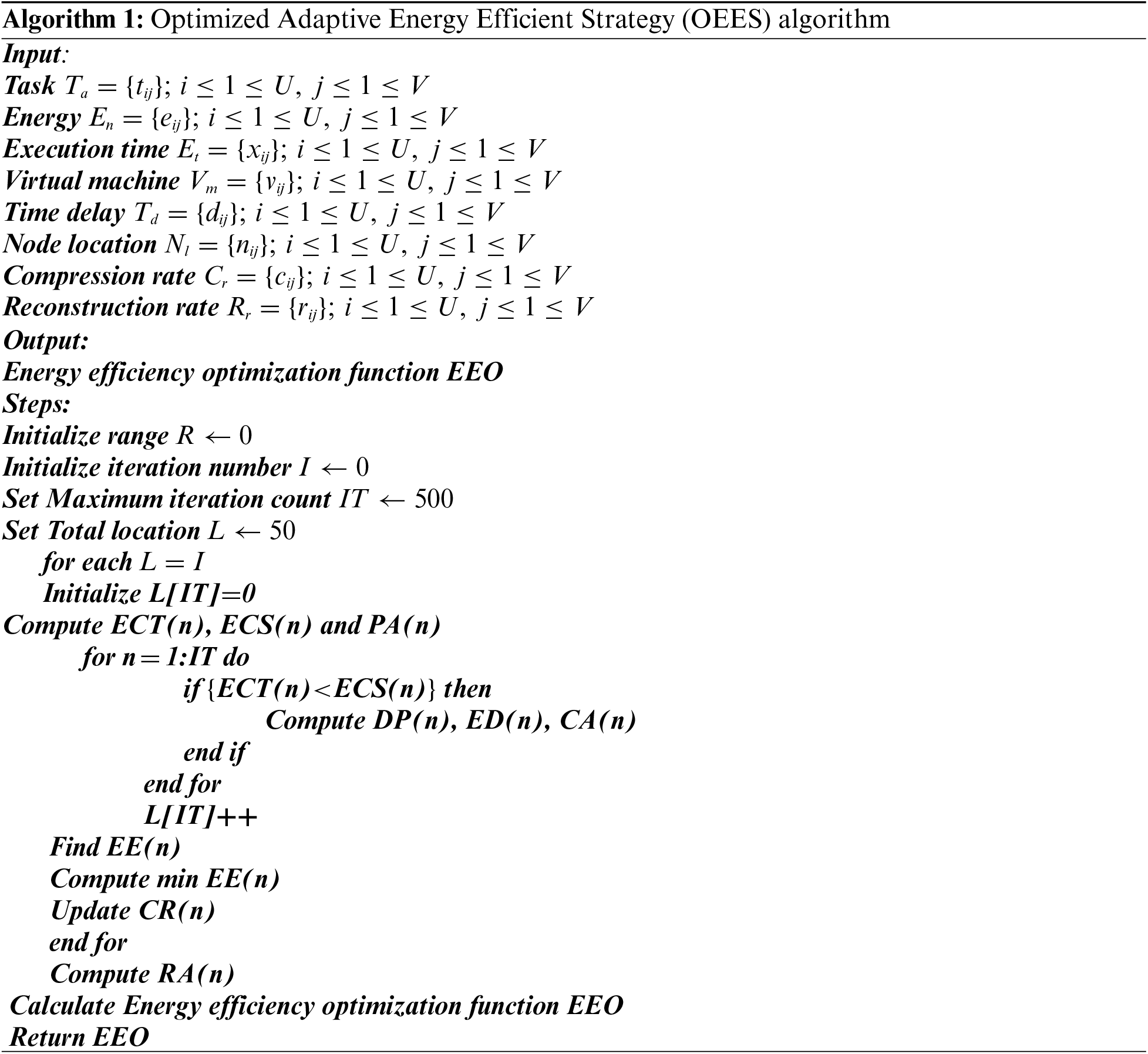Energy consumption is computed as

ECT(n)=i=1Uj=1V[tij+(eij)tijeij][xij+(vij)xijvij](1)

where, ECT(n) is the energy consumed, tij is the task set, eij is the energy set, xij is the execution time set, vij is the virtual machine set, (eij) is the rate of change of energy, (vij) is the rate of change of virtual machine, tij+(eij) is the sum of the task set and rate of change of energy and xij+(vij) is the sum of execution time set and rate of change of virtual machine. This value gives the amount of energy consumed by the virtual machine.

Energy conserved is computed as

ECS(n)=i=1Uj=1VECT(n)/[eij+exp(xij,vij)ECT(n)dij](2)

where, ECS(n) is the energy conserved, ECT(n) is the energy consumed, eij is the energy set, xij is the execution time set, vij is the virtual machine set, dij is the time delay set, U represents the total x variants, and V represents the total y variants. This value gives the amount of energy conserved using the data reduction technique.

Predictive accuracy is given by

PA(n)=argmaxi,jECT(n)exp(ECS(n))xijvijdijnij(3)

where, PA(n) is the predictive accuracy, ECS(n) is the energy conserved, ECT(n) is the energy consumed, xij is the execution time set, vij is the virtual machine set, dij is the time delay set and nij is the node location set. The predictive accuracy gives the rate at which the data is predicted correctly.

Data performance rate is defined as

DP(n)=[PA(n)+mod(ECS(n))]vijdij[ECS(n)+mod(PA(n))]nijcij(4)

where DP(n) is the data performance rate, PA(n) is the predictive accuracy, ECS(n) is the energy conserved, vij is the virtual machine set, dij is the time delay set, nij is the node location set and cij is the compression rate. The data performance rate is the rate at which the data is reconstructed.

Execution delay is computed as

ED(n)={PA(n)i=1Mdijnijdij+nij}/{i=1Mcijrijcij+rijDP(n)}(5)

where ED(n) is the execution delay, dij is the time delay set, nij is the node location set, cij is the compression rate, rij is the reconstruction rate, DP(n) is the data performance rate and PA(n) is the predictive accuracy. The execution delay is the time delay involved in the execution of virtual program.

Cloud accuracy is given by

CA(n)=i=1Ui=1V{exp(DP(n)nij)+exp(ED(n)cij)rij}(6)

CA(n) is the cloud accuracy, ED(n) is the execution delay, DP(n) is the data performance rate, nij is the node location set, cij is the compression rate and rij is the reconstruction rate. The cloud accuracy represents the rate at which the cloud data is processed correctly.

Edge efficiency is computed as

EE(n)=1UVi=1Uj=1V[||CA(n)ED(n)||22tijeij+||CA(n)ED(n)||22xijvij](7)

EE(n) is the edge efficiency, CA(n) is the cloud accuracy, ED(n) is the execution delay, tij is the task set, eij is the energy set, xij is the execution time set, vij is the virtual machine set and the term ||CA(n)ED(n)||22 is the difference between cloud accuracy and execution delay. The edge efficiency gives the efficiency of the edge network.

Compression ratio is calculated as

CR(n)=ij[(EE(n)eij)+(xijvij)]CA(n)dij(8)

where CR(n) is the compression ratio, EE(n) is the edge efficiency, CA(n) is the cloud accuracy, eij is the energy set, xij is the execution time set, vij is the virtual machine set, dij is the time delay set and (xijvij) is the product of execution time set and the virtual machine set. Compression ratio gives the rate at which the data is compressed using the compression algorithm.

Reconstruction accuracy is given by

RA(n)=i0maxx=i{dij,0}||CR(n)xij||22+vijnijEE(n)(9)

where RA(n) is the reconstruction accuracy, xij is the execution time set, vij is the virtual machine set, dij is the time delay set, max{dij,0} selects the maximum among zero and the time delay set. Reconstruction accuracy gives the rate at which the data is reconstructed efficiently.

Energy efficiency optimization function EEO

EEO=Minimizen=1NECT(n)ECS(n)DP(n)/ED(n)+f=1NCA(n)EE(n)PA(n)/CR(n)s.t.nRA(n)(10)

where EEO is the energy efficiency optimization function, ECS(n) is the energy conserved, ECT(n) is the energy consumed, DP(n) is the data performance rate, PA(n) is the predictive accuracy, EE(n) is the edge efficiency, CA(n) is the cloud accuracy, ED(n) is the execution delay, CR(n) is the compression ratio and RA(n) is the reconstruction accuracy.

5  Results and Discussion

In this section, we have performed the performance analysis of the proposed scheme. The proposed OEES scheme was compared using various algorithms like dictionary compression (DC), prefix encoding compression (PEC), indirect encoding compression (IEC), sparse encoding compression (SEC), cluster compression (CC) and run-length encoding compression (REC). All the simulations were performed using Intel core i5 processor using Virtual box VM execution environment in a system with 6GB RAM.

5.1 Performance Analysis

For quantitative evaluation we have employed metrics like energy consumption, energy conserved, predictive accuracy, data performance rate, execution delay, cloud accuracy, edge efficiency, compression ratio and reconstruction accuracy.

Tab. 1 shows the comparison of energy consumption for variation in the number of virtual machines (VMs). It is clearly observed that, the overall energy consumption for dictionary compression is 21.44 KJ, prefix encoding compression is 27.78 KJ, indirect encoding compression is 28.76 KJ, sparse encoding compression is 22.24 KJ, cluster compression is 20.01 KJ and run-length encoding compression is 23.68 KJ. The proposed scheme attains a minimal energy consumption of 7.38 KJ. This is due to the effective data compression scheme implemented in the proposed system.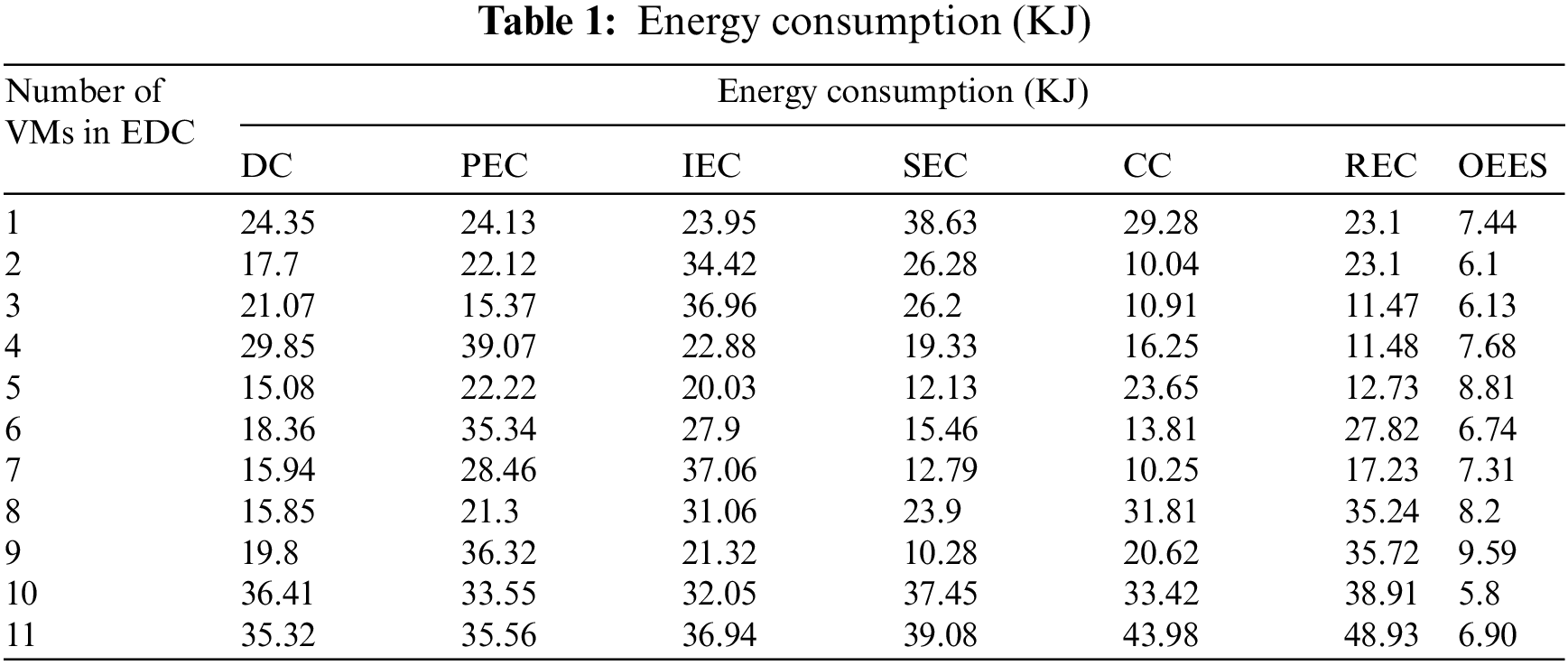Tab. 2 shows the comparison of energy conserved for variation in the number of virtual machines (VMs). It is clearly observed that, the overall energy conserved for dictionary compression is 14.92 KJ, prefix encoding compression is 15.14 KJ, indirect encoding compression is 15.23 KJ, sparse encoding compression is 16.15 KJ, cluster compression is 16.74 KJ and run-length encoding compression is 13.59 KJ. The proposed scheme attains a highest energy conservation of 55.57 KJ. This is because of the usage of measurement matrix in the IoT data reduction.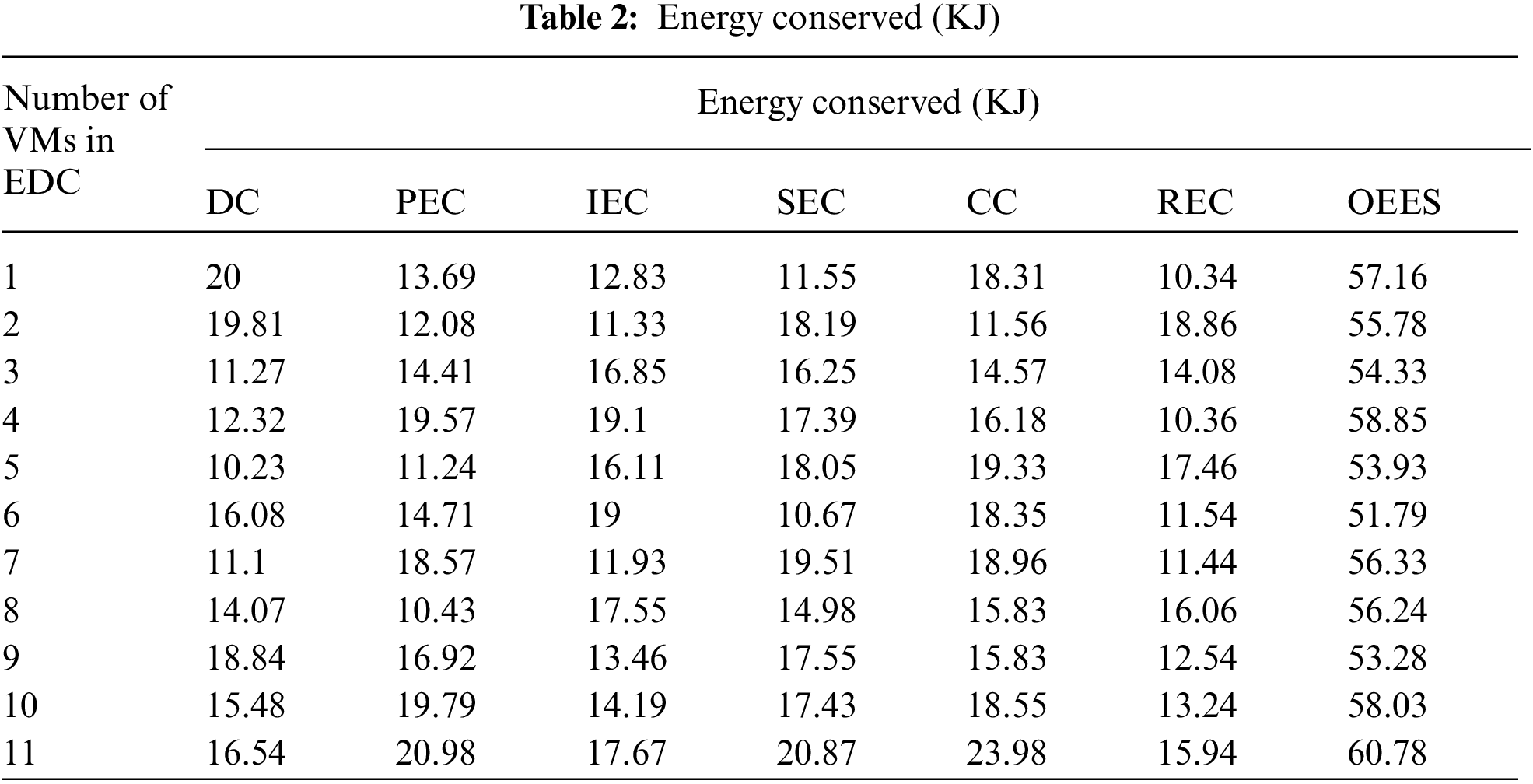Fig. 4 shows the comparison of execution delays for variation in the number of virtual machines (VMs). It is clearly observed that, the overall execution delay for dictionary compression is 51.67 ms, prefix encoding compression is 48.32 ms, indirect encoding compression is 60.73 ms, sparse encoding compression is 56.22 ms, cluster compression is 50.54 ms and run-length encoding compression is 47.48 ms. The proposed scheme attains a lowest execution delay of 14.49 ms. The least value of execution delay in this scheme is due to the incorporation of time delay factor in the optimization. The least value of execution delay in this scheme is due to incorporating the time delay factor in the optimization. The Internet of Things (IoT) benefits from computing power being located closer to where a physical device or data source exists. Sensor and device data must be analyzed at the edge rather than traveling back to the central site for analysis before it can be used to react faster or mitigate issues. Edge computing reduces communication latency between IoT devices and the central IT networks to which they are connected by processing and storing data locally.Figure 4: Variation of execution delay

Fig. 5 depict the variation of cloud accuracy. The cloud accuracy of the proposed OESS scheme is as high as 85.07%. However other schemes like dictionary compression, prefix encoding compression, indirect encoding compression, sparse encoding compression, cluster compression and run-length encoding compression have low cloud accuracy of 56.93%, 52.54%, 54.49%, 57.47%, 55.35% and 57.44% respectively. The cloud accuracy of the proposed scheme is high since it performs all the tasks in accurate manner using high reconstruction rate.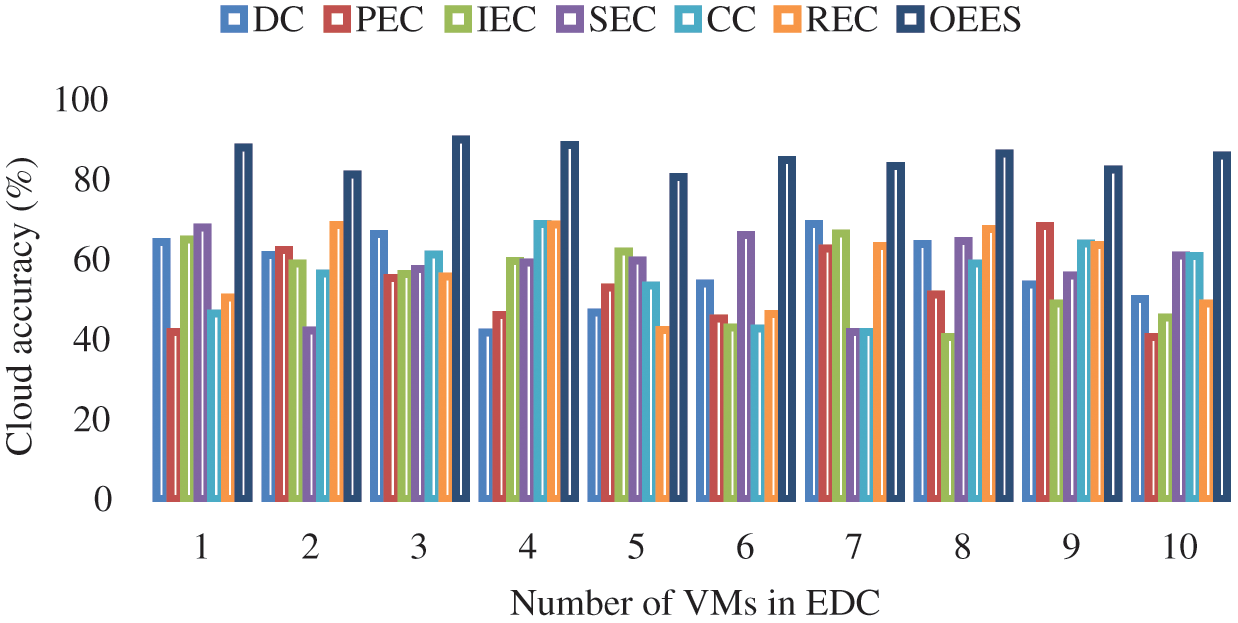Figure 5: Variation of cloud accuracy

Fig. 6 shows the variation of edge efficiency. The edge efficiency of the proposed OESS scheme is as high as 85.07%. However other schemes like dictionary compression, prefix encoding compression, indirect encoding compression, sparse encoding compression, cluster compression and run-length encoding compression have low edge efficiency of 46.19%, 47.70%, 41.3%, 47.13%, 45.48% and 44.14% respectively. The edge efficiency of the proposed scheme is high since all the edge devices employed in this framework have good prediction rate.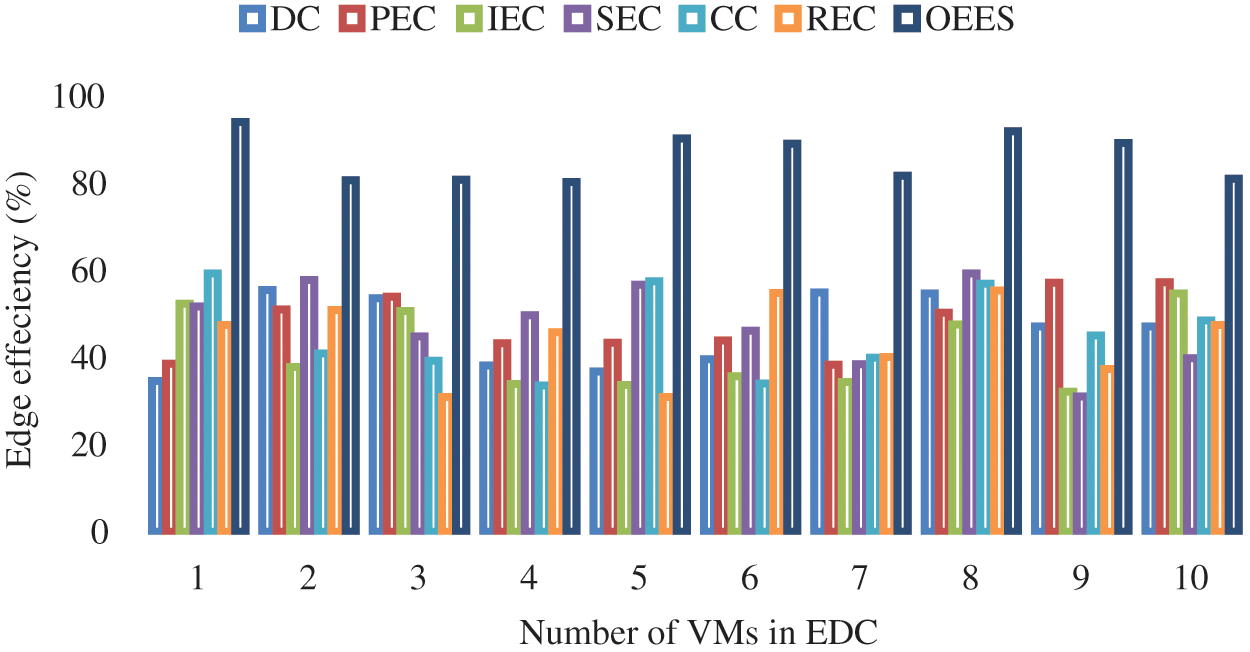Figure 6: Variation of edge efficiency

Fig. 7 shows the variation of compression ratio. The compression ratio of the proposed OESS scheme is as high as 85.82%. However other schemes like dictionary compression, prefix encoding compression, indirect encoding compression, sparse encoding compression, cluster compression and run-length encoding compression have low compression ratio of 43.21%, 44.20%, 44.3%, 49.13%, 45.93% and 41.94% respectively. The compression ratio of the proposed scheme is the highest since OEES scheme utilizes compression based on compressive sensing theory.Figure 7: Variation of compression ratio

Tab. 3 shows the comparison of predictive accuracy for variation in the number of virtual machines (VMs). It is clearly observed that, the overall predictive accuracy for dictionary compression is 31.48%, prefix encoding compression is 47.07%, indirect encoding compression is 45.05%, sparse encoding compression is 42.15%, cluster compression is 37.59% and run-length encoding compression is 45.66%. The proposed scheme attains a maximum predictive accuracy of 85.11%. The proposed OESS scheme has a prediction rate greater than 85% since it employs virtual machine for the energy computation.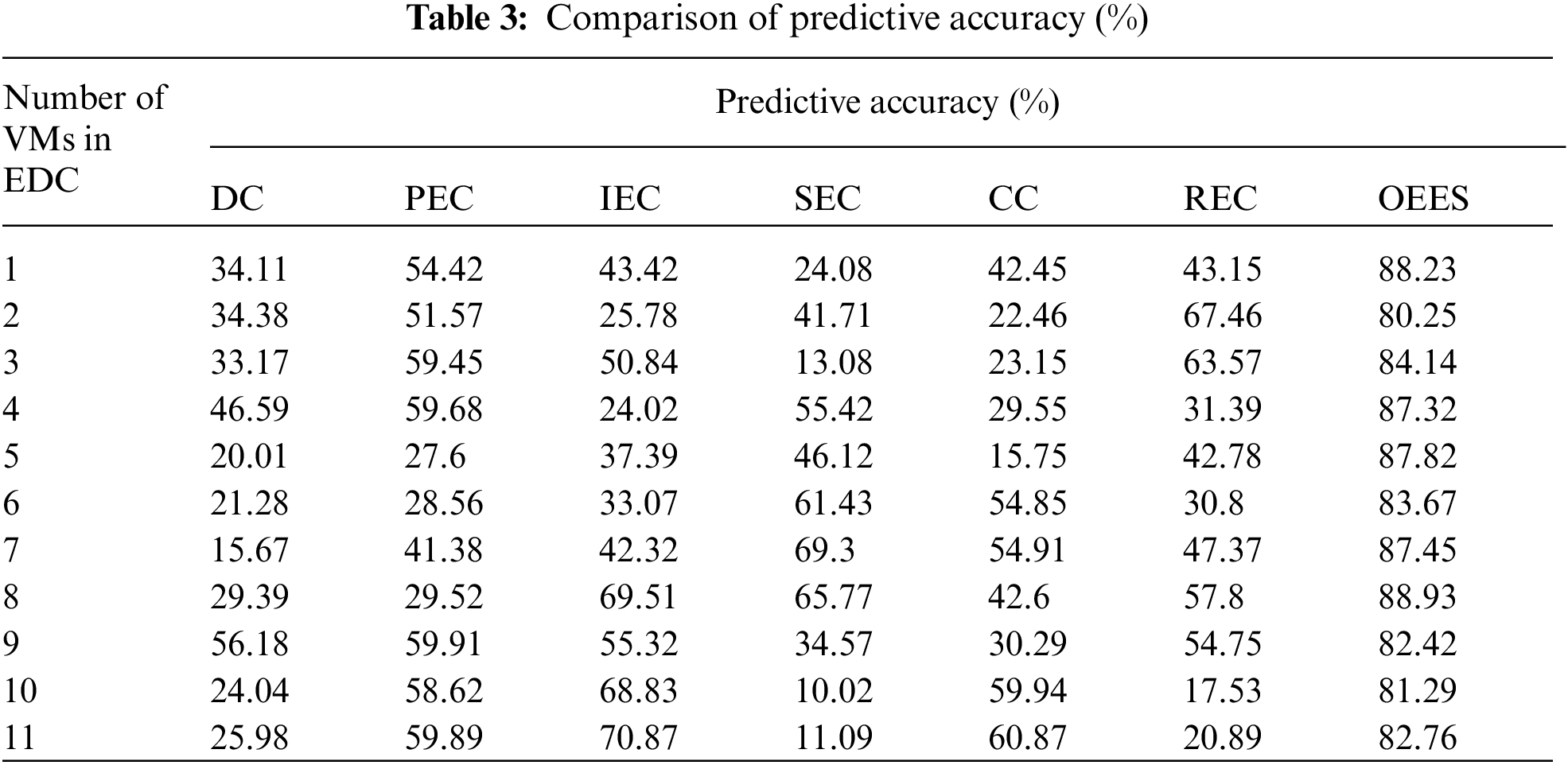Tab. 4 shows the variation of data performance rate. The data performance rate of the proposed OESS scheme is as high as 85.82%. However other schemes like dictionary compression, prefix encoding compression, indirect encoding compression, sparse encoding compression, cluster compression and run-length encoding compression have low data performance rate of 43.21%, 44.20%, 44.3%, 49.13%, 45.93% and 41.94% respectively. The data performance rate of the proposed scheme is as high as 85% since it uses minimal execution delay with highest energy conservation.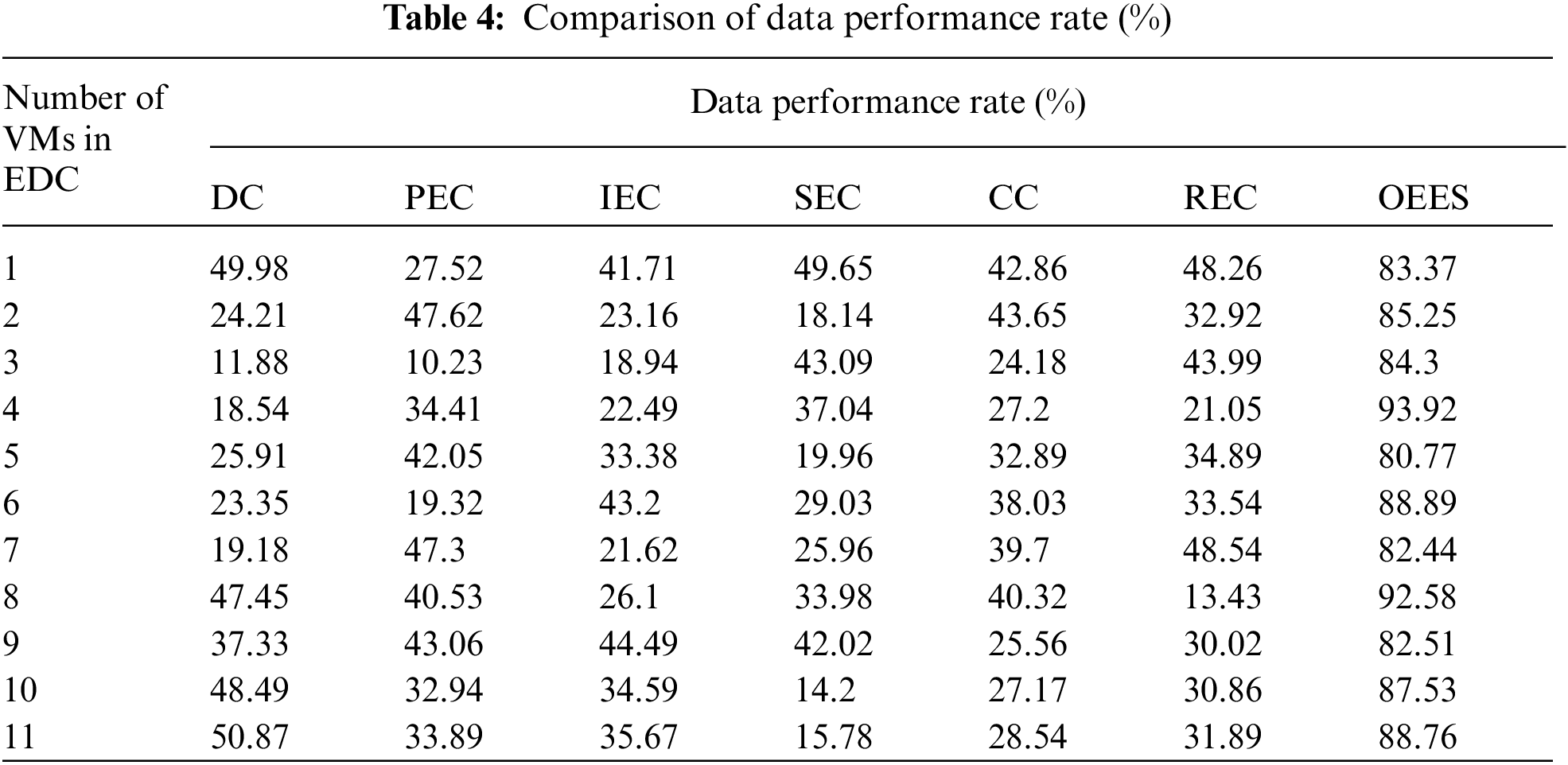6  Conclusion and Future Work

This research presented a new approach called Optimized Energy Efficient Strategy (OEES) for the optimal data transfer and data reduction between cloud devices. In this model, the acquisition of data from the cloud-based devices was done initially. This data was then displayed using the display modules. The displayed data was then stored for temporary usage using data storage devices. The stored data was encrypted using encryption algorithms. The encrypted data was then analyzed and compressed using the proposed OESS algorithm. The proposed OEES scheme was compared using various algorithms like dictionary compression, prefix encoding compression, indirect encoding compression, sparse encoding compression, cluster compression and run-length encoding compression. It was inferred that the proposed OESS scheme attained energy consumption of 7.38 KJ, energy conservation of 55.57 kJ, predictive accuracy of 97.5%, data performance rate of 97.65%, execution delay of 14.49 ms, cloud accuracy of 85.12%, edge efficiency of 85.07%, compression ratio of 85.82% and reconstruction accuracy of 80.04%. In future, we plan to develop a new application for the implementation of energy efficient data reduction in cloud data services. Edge computing reduces the amount of data flowing to and from the primary network by processing data closer to the source and prioritizing traffic. As a result, latency is reduced, and overall speed increases. Athletes need a lot of room to operate effectively. For industrial and enterprise-level businesses, edge computing is critical because it creates new and improved ways to maximize operational efficiency and safety while also improving performance and reliability.

Acknowledgement: The authors would like to thank for the support from Taif University Researchers Supporting Project number (TURSP-2020/98), Taif University, Taif, Saudi Arabia.

Funding Statement: Taif University Researchers Supporting Project number (TURSP-2020/98), Taif University, Taif, Saudi Arabia.

Conflicts of Interest: We declare that we have no conflicts of interest to report regarding the present study.

References

1.  1.  Z. Xiong, Y. Zhang, N. C. Luong, D. Niyato, P. Wang et al., “The best of both worlds: A general architecture for data management in blockchain-enabled internet-of-things,” IEEE Network, vol. 34, no. 1, pp. 166–173, 2020.
2.  2.  R. K. Singh, R. Berkvens and M. Weyn, “Energy efficient wireless communication for IoT enabled greenhouses,” in Proc. 2020 Int. Conf. on Communications Systems and Networks, COMSNETS 2020, Bangalore, India, pp. 885–887, 2020.
3.  3.  I. Sittón-Candanedo, R. S. Alonso, Ó. García, A. B. Gil and S. Rodríguez-González, “A review on edge computing in smart energy by means of a systematic mapping study,” Electron, vol. 9, no. 1, pp. 1–33, 2020.
4.  4.  P. P. Ray, D. Dash and D. De, “Edge computing for internet of things: A survey, e-healthcare case study and future direction,” Journal of Network & Computer Application, vol. 140, no. May, pp. 1–22, 2019.
5.  5.  M. Bendechache, S. Svorobej, P. T. Endo and T. Lynn, “Simulating resource management across the cloud-to-thing continuum: A survey and future directions,” Future Internet, vol. 12, no. 6, pp. 1–25, 2020.
6.  6.  W. Liu, G. Huang, A. Zheng and J. Liu, “Research on the optimization of IIoT data processing latency,” Computer Communication, vol. 151, no. January, pp. 290–298, 2020.
7.  7.  H. M. Al-Kadhim and H. S. Al-Raweshidy, “Energy efficient and reliable transport of data in cloud-based IoT,” IEEE Access, vol. 7, no. 5, pp. 64641–64650, 2019.
8.  8.  S. Aheleroff, “IoT-Enabled smart appliances under industry 4.0: A case study,” Advance Engineering Informatics, vol. 43, no. May 2019, pp. 101043, 2020.
9.  9.  A. Banijamali, O. P. Pakanen, P. Kuvaja and M. Oivo, “Software architectures of the convergence of cloud computing and the internet of things: A systematic literature review,” Informationand Software Technology, vol. 122, no. November 2018, pp. 106271, 2020.
10. 10. M. Krichen, S. Mechti, R. Alroobaea, E. Said, P. Singh et al., “A formal testing model for operating room control system using internet of things,” Computers,” Materials & Continua, vol. 66, no. 3, pp. 2997–3011, 2021.
11. 11. M. Chen, W. Li, G. Fortino, Y. Hao, L. Hu et al., “A dynamic service migration mechanism in edge cognitive computing,” ACM Transaction on Internet Technology, vol. 19, no. 2, pp. 1–15, 2019.
12. 12. M. Masud, G. S. Gaba and K. Choudhary,“A robust and lightweight secure access scheme for cloud based E-healthcare services,”. Peer-to-Peer Networking and Applications, vol. 14, pp. 3043–3057, 2021.
13. 13. M. H. Shahid, A. R. Hameed, S. ul Islam, H. A. Khattak, I. U. Din et al., “Energy and delay efficient fog computing using caching mechanism,” Computer Communications, vol. 154, no. October 2019, pp. 534–541, 2020.
14. 14. Z. Zhou, S. Yu, W. Chen and X. Chen, “CE-IoT: Cost-effective cloud-edge resource provisioning for heterogeneous IoT applications,” IEEE Internet Things Journal, vol. 7, no. 9, pp. 8600–8614, 2020.
15. 15. A. Javed, J. Robert, K. Heljanko and K. Främling, “IoTEF: A federated edge-cloud architecture for fault-tolerant IoT applications,” Journal of Grid Computing, vol. 18, no. 1, pp. 57–80, 2020.
16. 16. I. Khan, X. Tao, G. M. Shafiqur Rahman, W. U. Rehman and T. Salam, “Advanced energy-efficient computation offloading using deep reinforcement learning in MTC edge computing,” IEEE Access, vol. 8, no. 4, pp. 82867–82875, 2020.
17. 17. X. Wang, Y. Han, V. C. M. Leung, D. Niyato, X. Yan et al., “Convergence of edge computing and deep learning: A comprehensive survey,” IEEE Communications Surveys & Tutorials, vol. 22, no. 2, pp. 869–904, 2020.
18. 18. C. Jiang, “Energy aware edge computing: A survey,” Computer Communications, vol. 151, no. 2, pp. 556–580, 2020.
19. 19. M. S. Mekala and P. Viswanathan, “A survey: Energy-efficient sensor and VM selection approaches in green computing for X-IoT applications,” International Journal of Computers and Applications, vol. 42, no. 3, pp. 290–305, 2020.
20. 20. Y. Shi, K. Yang, T. Jiang, J. Zhang and K. B. Letaief, “Communication-efficient edge AI: Algorithms and systems,” IEEE Communications Surveys and Tutorials, vol. 22, no. 4, pp. 2167–2191, 2020.
21. 21. B. C. Kavitha, R. Vallikannu and K. S. Sankaran, “Delay-aware concurrent data management method for IoT collaborative mobile edge computing environment,” Microprocessors and Microsystems, vol. 74, no. 4, pp. 103021, 2020.
22. 22. H. Zahmatkesh and F. Al-Turjman, “Fog computing for sustainable smart cities in the IoT era: Caching techniques and enabling technologies - an overview,” Sustainable Cities and Socities, vol. 59, no. January, pp. 102139, 2020.
23. 23. H. Rimminen, J. Lindström, M. Linnavuo and R. Sepponen, “Detection of falls among the elderly by a floor sensor using the electric near field,” IEEE Transaction on Information Technology in Biomedeicine, vol. 14, no. 6, pp. 1475–1476, 2010.
24. 24. I. Mohiuddin and A. Almogren, “Security challenges and strategies for the IoT in cloud computing,” in Proc. Int. Conf. on Information and Communication Systems, (ICICS 2020), Irbid, Jordan, pp. 367–372, 2020.
25. 25. J. Mades, G. Ebelt, B. Janjic, F. Lauer, C. C. Rheinlander et al., “TLS-Level security for Low power industrial IoT network infrastructures,” in Proc. 2020 Design, Automation & Test in Europe Conf. and Exhibiton, DATE 2020, Grenoble, France, pp. 1720–1721, 2020.
26. 26. R. Kiruthiga and D. Akila, “Prediction-based cost-efficient resource allocation scheme for Big data streams in cloud systems,” in Proc. Int. Conf. on Mathematical Modeling and Computational Science: ICMMCS, Pattaya, Thailand, pp. 233–242, 2020.
27. 27. M. Masud, G. S. Gaba, K. Choudhary, M. S. Hossain, M. F. Alhamid and G. Muhammad, “Lightweight and anonymity-preserving user authentication scheme for IoT-based healthcare,” in IEEE Internet of Things Journal, DOI 10.1109/JIOT.2021.3080461.
28. 28. S. Jeyalaksshmi, M. S. Nidhya, G. Suseendran, S. Pal and D. Akila, “Developing mapping and allotment in volunteer cloud systems using reliability profile algorithms in a virtual machine,” in Proc. 2nd IEEE Int. Conf. on Computation, Automation and Knowledge Management, Dubai, UAE, pp. 97–101, 2021.
29. 29. S. Pal, and P. K. Pattnaik, “A Simulation-based approach to optimize the execution time and minimization of average waiting time using queuing model in cloud computing environment,” International Journal of Electrical and Computer Engineering, vol. 6, no. 2, pp. 743–750, April 2016.
30. 30. S. Pal, R. Kumar, L. H. Son, K. Saravanan, M. Abdel-Basset et al., “Novel probabilistic resource migration algorithm for cross-cloud live migration of virtual machines in public cloud,” Journal of Supercomputing, vol. 75, no. May, pp. 5848–5865, 2019.This work is licensed under a Creative Commons Attribution 4.0 International License, which permits unrestricted use, distribution, and reproduction in any medium, provided the original work is properly cited.